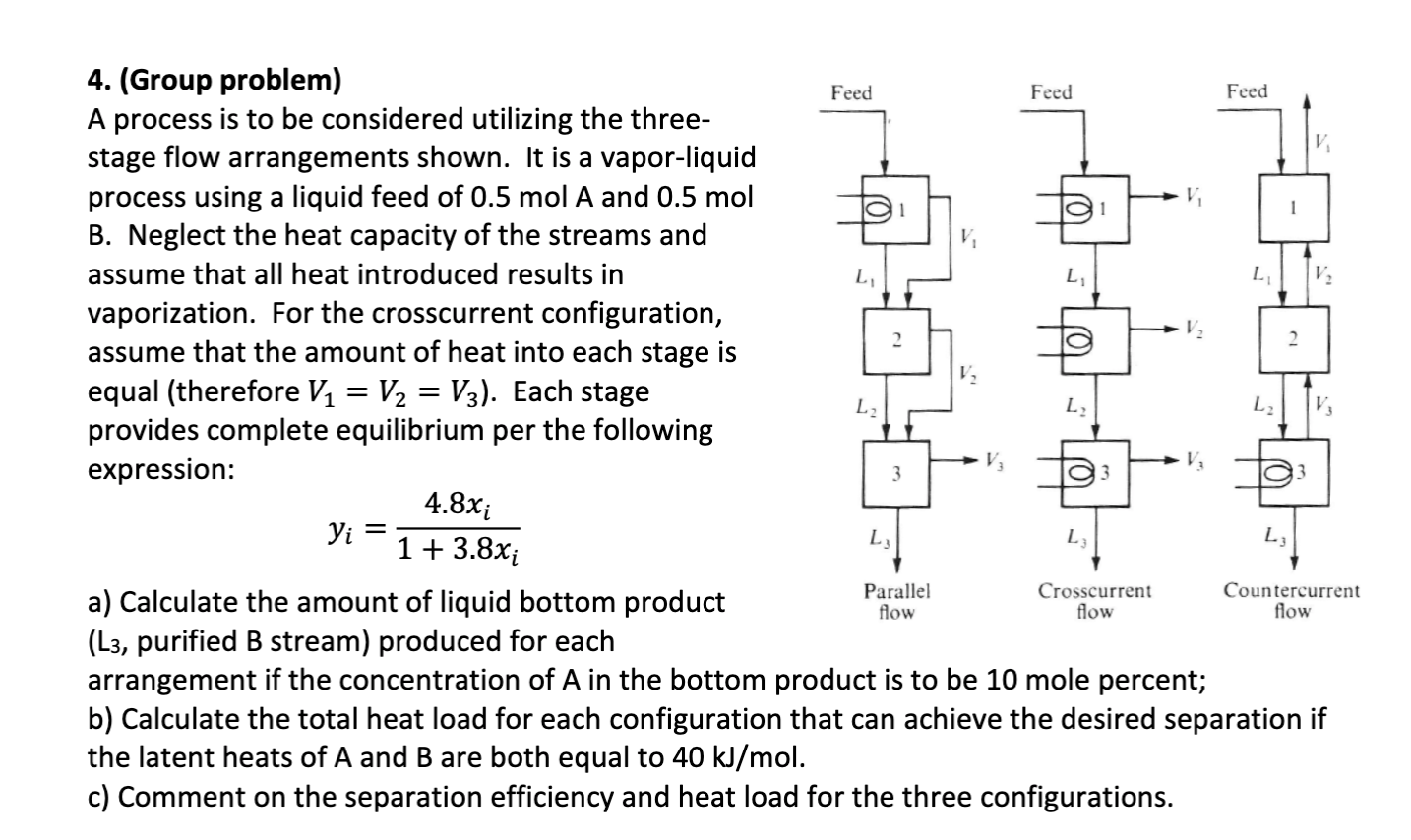Home / Answered Questions / Other / feed-feed-feed-4-group-problem-a-process-is-to-be-considered-utilizing-the-three-stage-flow-arrangem-aw351

# (Solved): Feed Feed Feed 4. (Group Problem) A Process Is To Be Considered Utilizing The Three- Stage Flow Arra...Feed Feed Feed 4. (Group problem) A process is to be considered utilizing the three- stage flow arrangements shown. It is a vapor-liquid process using a liquid feed of 0.5 mol A and 0.5 mol B. Neglect the heat capacity of the streams and assume that all heat introduced results in vaporization. For the crosscurrent configuration, assume that the amount of heat into each stage is equal (therefore V1 = V2 = 13). Each stage provides complete equilibrium per the following expression: 4.8xi --- Yi = 1 + 3.8Xi L L Parallel flow Crosscurrent flow Countercurrent flow a) Calculate the amount of liquid bottom product (L3, purified B stream) produced for each arrangement if the concentration of A in the bottom product is to be 10 mole percent; b) Calculate the total heat load for each configuration that can achieve the desired separation if the latent heats of A and B are both equal to 40 kJ/mol. c) Comment on the separation efficiency and heat load for the three configurations.

We have an Answer from Expert